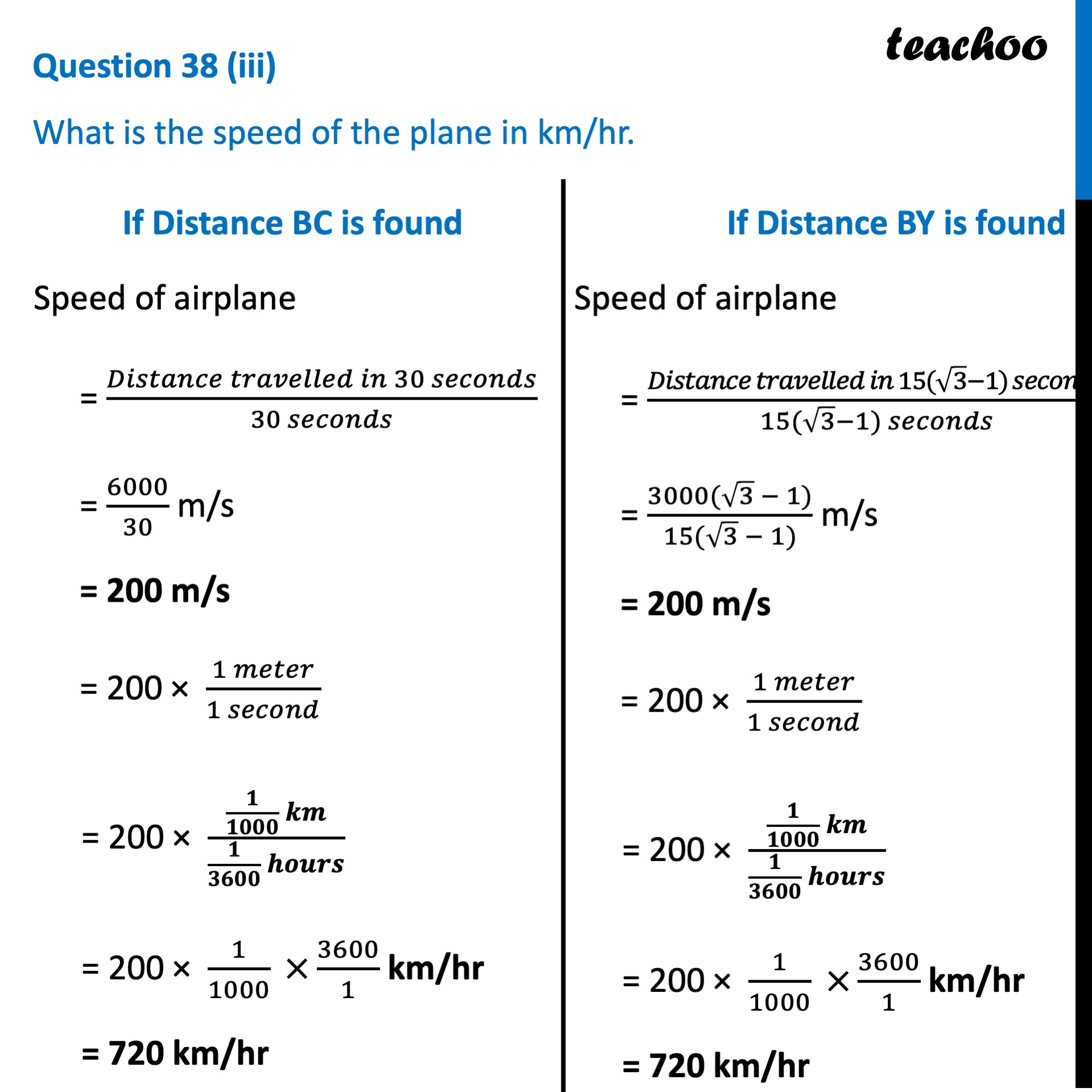CBSE Class 10 Sample Paper for 2023 Boards - Maths Standard

Class 10
Solutions of Sample Papers for Class 10 Boards

## What is the speed of the plane in km/hr.Learn in your speed, with individual attention - Teachoo Maths 1-on-1 Class

### Transcript

Question 38 (iii) What is the speed of the plane in km/hr. If Distance BC is found Speed of airplane = (𝐷𝑖𝑠𝑡𝑎𝑛𝑐𝑒 𝑡𝑟𝑎𝑣𝑒𝑙𝑙𝑒𝑑 𝑖𝑛 30 𝑠𝑒𝑐𝑜𝑛𝑑𝑠)/(30 𝑠𝑒𝑐𝑜𝑛𝑑𝑠) = 6000/30 m/s = 200 m/s = 200 × (1 𝑚𝑒𝑡𝑒𝑟)/(1 𝑠𝑒𝑐𝑜𝑛𝑑) = 200 × (𝟏/𝟏𝟎𝟎𝟎 𝒌𝒎)/(𝟏/𝟑𝟔𝟎𝟎 𝒉𝒐𝒖𝒓𝒔) = 200 × 1/1000 ×3600/1 km/hr = 720 km/hr If Distance BY is found Speed of airplane = (𝐷𝑖𝑠𝑡𝑎𝑛𝑐𝑒 𝑡𝑟𝑎𝑣𝑒𝑙𝑙𝑒𝑑 𝑖𝑛 15(√3−1) 𝑠𝑒𝑐𝑜𝑛𝑑𝑠)/(15(√3−1) 𝑠𝑒𝑐𝑜𝑛𝑑𝑠) = (3000(√3 − 1))/(15(√3 − 1)) m/s = 200 m/s = 200 × (1 𝑚𝑒𝑡𝑒𝑟)/(1 𝑠𝑒𝑐𝑜𝑛𝑑) = 200 × (𝟏/𝟏𝟎𝟎𝟎 𝒌𝒎)/(𝟏/𝟑𝟔𝟎𝟎 𝒉𝒐𝒖𝒓𝒔) = 200 × 1/1000 ×3600/1 km/hr = 720 km/hr